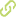# A statistical and comparative study of quantum walks under weak measurements and weak values regimes•
• Overview
•
• Identity
•
•
• View All
•

### Abstract

• Quantum walks have been studied under several regimes. Motivated by experimental results on quantum weak measurements and weak values as well as by the need to develop new insights for quantum algorithm development, we are extending our knowledge by studying the behavior of quantum walks under the regime of quantum weak measurements and weak values of pre- and postselected measurements (QWWM hereinafter). In particular, we investigate the limiting position probability distribution and several statistical measures (such as standard deviation) of a QWWM on an infinite line, and compare such results with corresponding classical and quantum walks position probability distributions and statistical measures, stressing the differences provided by weak measurements and weak values with respect to results computed by using canonical observables. We start by producing a concise introduction to quantum weak values and quantum weak measurements. We then introduce definitions as well as both analytical and numerical results for a QWWM under Hadamard evolution and extend our analysis to quantum evolution ruled by general unitary operators. Moreover, we propose a definition and focus on the properties of mixing time of QWWM on an infinite line, followed by a comparison of known corresponding results for classical and quantum walks mixing times. We finish this paper by presenting a plausible experimental implementation of a QWWM.

### Publication date

• July 21, 2011